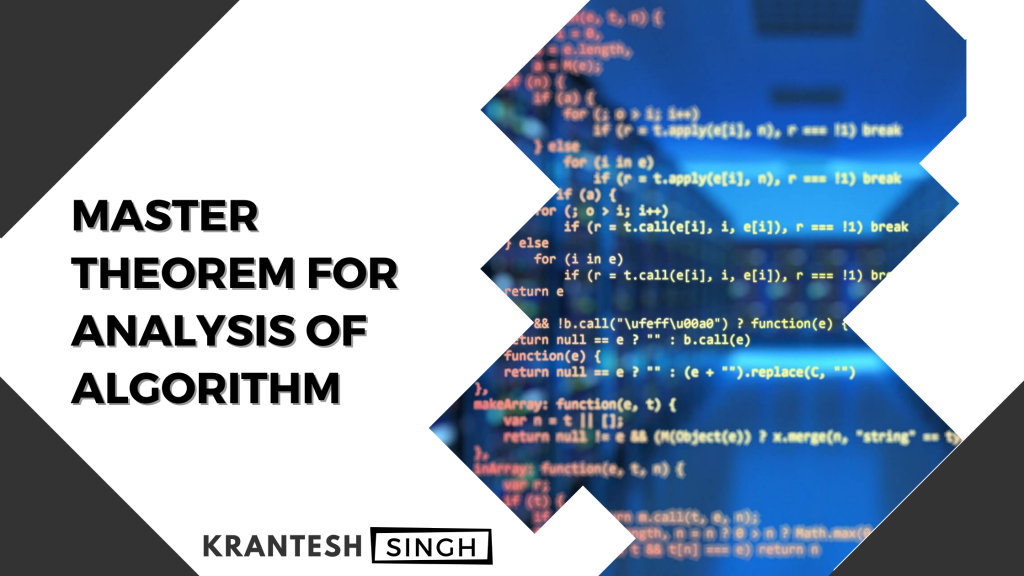Krantesh Singh

# Programming## Master Theorem for Analysis of Algorithm

If you’re a computer science student or a programmer you may be familiar with term algorithm and time complexity related to a computer program so in this article you’re going to learn about MASTER THEOREM – Analysis of Algorithm. We use master theorem for given recursive equation. If we have recursive equation then by using …